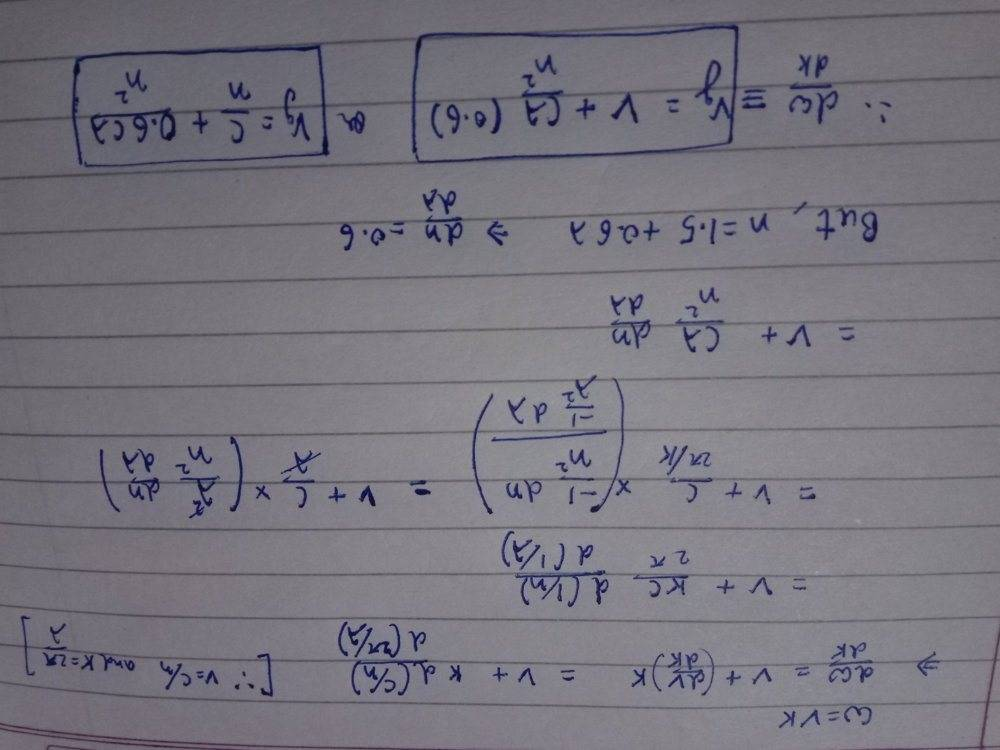# Group velocity and the dispersion relation

Kaguro
Homework Statement:
Q.Light of wavelength λ (in free space) propagates through a dispersive medium with refractive index n(λ)=1.5 + 0.6λ. The group velocity of a wave travelling inside this medium in units of 10^8 m/s is
(A) 1.5 (B) 2.0 (C) 3.0 (D)4.0
Relevant Equations:
Group velocity is dw/dk. And phase velocity v is w/k.
After noting w=vk and differentiating with respect to k, and lots of simplifying, I get:

Vg = c/n +(2*pi*0.6)/(k*n)

This doesn't correspond to any numerical value though...

Homework Helper
Gold Member
You differentiated what with respect to k?

Kaguro
You differentiated what with respect to k?
I differentiated both sides of the equation w=vk w.r.t k

This will give me
dw/dk=v + k(dv/dk)

Group velocity is then dw/dk.
v is c/n the phase velocity..

and then I wrote k=2*pi/lamda
And substituted value of dk.

Homework Helper
Gold Member
It looks like you did not apply the chain rule correctly.

Last edited:
Kaguro
It looks like you did not apply the chain rule correctly.
Oh! That's right...

Now the value I get for group velocity is:

Vg=(c/n)+0.6*c*lamda/n^2

Is this correct?

Even so, this is not a numerical answer..

Homework Helper
Gold Member
Oh! That's right...

Now the value I get for group velocity is:

Vg=(c/n)+0.6*c*lamda/n^2

Is this correct?

Even so, this is not a numerical answer..
Look at your expression. It says that group velocity is equal to phase velocity plus something greater than zero. Can the group velocity be greater than the phase velocity? How did you get your answer. Please post the details of your work.

KaguroThis is my work. Sorry for not typing all this out. That would have taken me hours..